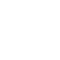# Flood-Peak Estimation

## Flood-Peak Estimation

A flood at an unusually high stage in a river, normally the level at which the river overflows its banks and inundates the adjoining area. The design of bridges, culvert waterways, and spillways for dams and estimation of the score at a hydraulic structure are some examples wherein flood-peak values are required. To estimate the magnitude of a flood, peak the following alternative methods are available:

1. Rational method
2. Empirical method
3. unit-hydrograph technique
4. Flood- frequency studies

### 1. Rational Method

The most realistic way to use the Rational Method is to consider it as a statistical link between the frequency distribution of rainfall and runoff. As such, it provides a means of estimating the design flood of a certain return period, with the rainfall duration equal to the time of concentration

If tp ≥ tc

Where,

Qp = Peak discharge in m3/sec

PC = Critical design rainfall in cm/hr

A = Area catchment in hectares

K = Coefficient of runoff.

Tp= Duration of rainfall

tc = Time of concentration

### 2. Empirical Formulae

(a) Dickens Formula (1865)

Where,

Qp = Flood peak discharge in m3/sec

A = Catchment area in km2.

CD = Dickens constant, 6 ≤ CD ≤ 30.

#### (b) Ryve’s formula (1884)

Where,

CH = Ryve’s constant

= 8.8 for the constant area within 80 km from the cost.

= 8.5 if the distance of area is 80 km to 160 km from the cost.

= 10.2 if area is Hilley and away from the cost.

#### (c) Inglis Formula (1930)

Where, A = Catchment area in Km2.

QP = Peak discharge in m3/sec.

## Flood Frequency Studies

(i) Recurrence interval or return Period:

where P = Probability of occurrence

(ii) Probability if non-occurrence: q = 1-P

(iii) Probability of an event occurring r times in ‘n’ successive years:

(iv) Reliability: (probability of non-occurrence /Assurance) = qn

(v) Risk = 1-qn

= 1(1-P)n

(vi) Safety Factory =

(vii) Safety Margin = Design value of the hydrological parameter – Estimated value of the hydrological parameter

## Gumbel’s Method

The extreme value distribution was an introduction by Gumbel (1941) and is commonly known as Gumbel’s distribution. it is one of the most widely used probability distribution functions for extreme values in hydrologic and meteorological studies for prediction of flood peaks, maximum rainfall, maximum wind speed.

Gumbel defined a flood as the largest of the 365 daily flows and the annual series of flood flows constitute a series of largest values of flows.

Where, XT = Peak value of hydrologic data

K = Frequency factor

yT = Reduced variate

T = Recurrence interval in year

yn = Reduced mean = 0.577

Sn = Reduced standard deviation.

Sn = 1.2825 for N → ∞

## Confidence Limit

Since the value of the variate for a given return period, xT determined by Gumbel’s method can have errors due to the limited sample data used. An estimate of the confidence limits of the estimates is desirable the confidence interval indicates the limits about the calculated value between which the true value can be said to lie with specific probability based on sampling errors only.

For a confidence probability c, the confidence interval of the variate xT is bounded by value x1 and x2 given by

Where, f(c) is a function of confidence probability ‘C’.

Se = Probability error

Where, N = Sample size

B = factor

σ = Standard deviation

## RECENT POST-

1.Bhavika says:
2.MPPSC Online Coaching Classes says: